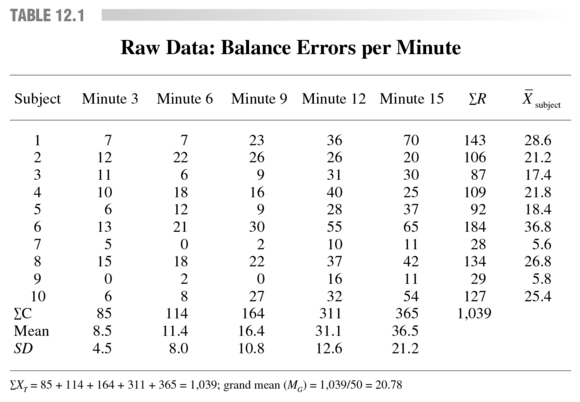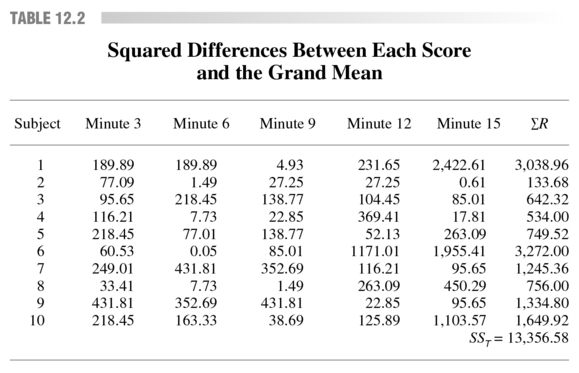Feedback

# Analyze variance of repeated measures with bicycle racing case study

### This is an excerpt from Statistics in Kinesiology-4th Edition by William Vincent & Joseph Weir.

Calculating Repeated Measures ANOVA

To demonstrate how to calculate ANOVA with repeated measures, we analyze a hypothetical study. A graduate student studying motor behavior was interested in the decrease in balance ability that bicycle racers experience as their fatigue increases. To measure this, the researcher placed a racing bicycle on a roller ergometer. A 4-inch-wide stripe was painted in the middle of the front roller, and the rider was required to keep the front wheel on the stripe. Balance was indicated by wobble in the front wheel and was measured by counting the number of times per minute that the front wheel of the bike strayed off the 4-inch stripe over a 15-minute test period.

As the test progressed, physiological fatigue increased and it became more and more difficult to maintain the front wheel on the stripe. The 15-minute test period was divided into five 3-minute periods for the purpose of collecting data. Data were collected on the number of balance errors during the last minute of each 3-minute period. In this design, the dependent variable is balance errors and the independent variable is time period (we call this variable &ldquo;time&rdquo;), which reflects the increase in fatigue.

Table 12.1 presents the raw data in columns and rows. The data (in errors per minute) for the subjects (N = 10) are in the rows, and the data for time (k = 5 repeated measures) are in the columns. The sum of rows (&sum; R) is the total score for each subject over all five time periods, and Xsubjects at the right denotes the mean across time for each subject. The sum of each column (&sum; C) is the total for all 10 subjects on a given trial; &sum; XT is presented at the bottom of the table.

Remember that ANOVA stands for analysis of variance. We analyze the variance by breaking the total variance in the data set into the relevant pieces. For the repeated measures ANOVA, we partition the total variance into pieces attributed to (1) differences between measurement periods [for the example in table 12.1, these are the differences between time periods (columns) at minute 3 and minute 6 and so on] , which is represented by how the means across time differ; (2) differences between subjects; and (3) unexplained variance (the error or residual). Notice that in contrast to the between-subjects ANOVA presented in chapter 11, where we could partition the total variance into only two pieces [between groups and within groups (error)] , in repeated measures ANOVA we have added a third piece. The third piece is the component attributable to differences between subjects. Because each subject provides a score for each time period, we can estimate how much of the total variance is due simply to different abilities of different subjects. As noted previously, in between-subjects ANOVA, interindividual differences are lumped into the error term.In repeated measures ANOVA, we partition variance by calculating the sums of squares (SS) for each piece so that

SStotal = SStime + SSsubjects + SSerror. (12.01)

We then divide each sum of squares by the appropriate degrees of freedom, which results in mean square values for each piece.

First, the total sums of squares (SST) is calculated by subtracting each score from the grand mean (MG), squaring the differences, and then adding the squared differences:

SST= &sum; [(Xi &minus; MG)2] . (12.02)

From table 12.1, we can see that the sum of all 50 scores (10 subjects times five time periods) is 1,039, resulting in a grand mean of 20.78. Table 12.2 shows the squared differences between each score and the grand mean.

For example, the score for subject one at minute 3 is 7 errors, and therefore the squared difference is (7 &minus; 20.78)2 = 189.88. By adding all the squared differences together, the resulting total sum of squares is 13,356.58.

To assess the variance due to differences between time periods, we must calculate the sum of the squared differences between each time period mean and the grand mean (with an adjustment for sample size):

SStime = n &sum; [(X-time &minus; MG)2] , (12.03)

where n is number of subjects. For the data in table 12.1, SStime = 10 [(8.5 &minus; 20.78)2 + &hellip; + (36.5 &minus; 20.78)2] = 6,115.88.To assess the variance due to differences between subjects, we subtract the mean for each subject across time from the grand mean, square these differences, add them up, and then multiply this sum by the number of trials:

SSsubjects = T &sum; (X-subject &minus; MG)2, (12.04)

where T is number of time periods. For the data in table 12.1, SSsubjects = 5 [(28.6
&minus; 20.78)2 + &hellip; + (25.4 &minus; 20.78)2] = 4,242.58.

To calculate the unexplained variance (SSerror ), we can rearrange equation 12.01 so that

SSerror = SStotal &minus; SStime &minus; SSsubjects. (12.05)

For our example, SSerror = 13,356.58 &minus; 6,115.88 &minus; 4,242.58 = 2,998.12.

We now have the necessary sums of squares values for the repeated measures ANOVA, but as in the between-subjects ANOVA in chapter 11, we need to divide the sums of squares values by their appropriate degrees of freedom. The degrees of freedom for time is

dftime = T &minus; 1. (12.06)

For the data in table 12.1, dftime = T &minus; 1 = 5 &minus; 1 = 4.

For the subjects effect, the degrees of freedom are calculated as

dfsubjects = N &minus; 1. (12.07)

In our example, dfsubjects = 10 &minus; 1 = 9.

For the error term, the degrees of freedom are calculated as

dferror = (T &minus; 1)(N &minus; 1). (12.08)

In our example, dferror = (5 &minus; 1)(10 &minus; 1) = 36.

Our next step is to calculate the mean square (MS) terms for each component. Recall from chapter 11 that a mean square value is the ratio of the sums of squares term divided by the appropriate df value. The mean square for time is calculated
as

MStime = SStime/dftime. (12.09)

For the data in table 12.2, MStime = 6,115.88/4 = 1,528.97.

The mean square for subjects is calculated as

MSsubjects = SSsubjects/dfsubjects. (12.10)

In our example, MSsubjects = 4,242.58/9 = 471.40.

Finally, the mean square error term is calculated as

MSerror = SSerror/dferror. (12.11)

In our example, MSerror = 2,998.12/36 = 83.28. We now have all the necessary pieces needed for the repeated measures ANOVA.

Recall from chapter 11 that an F ratio is a ratio of mean squares. The F ratio of interest is the F ratio for the time effect, which assesses whether the trial means differ. The F for time is calculated as

Ftime = MStime/MSerror. (12.12)

For our example, Ftime = 1,528.97/83.28 = 18.36 (see table 12.3).

It is also possible to calculate an F value for subjects (Fsubjects = MSsubjects/MSerror). But this value is not of interest at this time because it simply represents a test of the variability among the subjects. This Fsubjects value is not important to the research question being considered (Does an increase in fatigue result in an increase in mean balance errors across time?). We use Fsubjects in chapter 13 when intraclass correlation for reliability is discussed.

To determine the significance of F for time, we look in tables A.4, A.5, and A.6 of appendix A. The dftime is the same as dfB from chapter 11. The dferror is used in the same way as dfE in chapter 11&mdash;to measure within-group variability. Table A.6 shows that for df (4, 36) an F of 4.02 is needed to reach significance at &alpha; = .01. Because our obtained F (18.36) easily exceeds 4.02, we conclude that differences do exist somewhere among the mean values for the five time periods
at p &lt; .01.

Read more from Statistics in Kinesiology, Fourth Edition, by William Vincent and Joseph Weir.